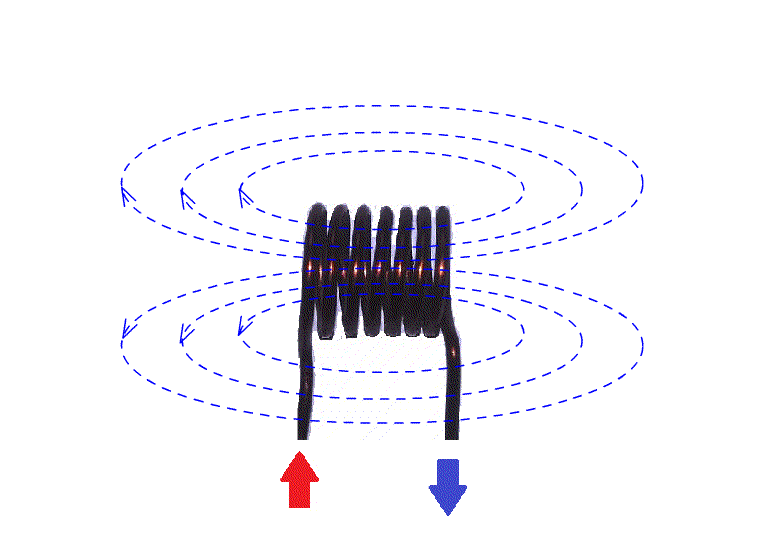top of page

## Inductors (Coils):

Inductor is an electric conductor in coil form (shape), which generates self inductance due to change in direction of flow of current through it.

The property of inductor is inductance ( denoted by L in formulae) , which is measured in (units) Henrys. Since Henry is big unit, milli Henry (mH) is used in general## Inductance:

One Henry is defined as generating one volt by changing direction of one Ampere current in the coil at a rate of once per a second.

A magnetic field is produced in the coil due to change in direction of flow of current through the conductor and opposes the cause producing it may be called as Self Inductance. Choke used in a Tube-light set is a practical example for self inductance.

In case, the magnetic field produced by self inductance is effecting another coil surrounded by the first coil and producing voltage across the second coil, then is called Mutual Inductance. Transformer works on Mutual Inductance concept.## Inductor in DC circuits:

Since the inductor works on change of direction of current flow through its conductor, there is no effect of inductance, if a constant uni-direction current flows through it.

So, it has no effect in DC Circuits, except resistance caused by the conductor, which depends on the material property of the conductor.

One of the main use of Inductor (coil) in DC circuits is to prevent any signal or noise disturbance in DC line, if connected in series.

## Inductor in AC circuits:

Inductors are highly used in AC circuits due to its self inductance with various frequencies.

As the frequency passing through the Inductor increases, it offers more resistance due to opposing nature of cause, which produces it.

The resistance offered in AC signal across a Inductor (coil) is called Impedence marked as Z. So, for DC signal or power supply, a coil offers Zero impdence.

## Inductor - Mini Size:

Now-a-days inductors (coils) are available as Concealed coils. Either the inductance value is marked on the surface or Colour band code used to identify the inductance value. The colour code is followed as per resistor color code and the units are milli Henrys (mH).

Two models of concealed inductors are shown here.  These inductors are easy to fix on PCBs.bottom of page